# CBSE Class 12 Physics HOTs Electronic Devices Set B

## Chapter 14 Semiconductor Electronics Materials Devices and Simple Circuits Class 12 Physics HOTS

Class 12 Physics students should refer to the following high order thinking skills questions with answers for Chapter 14 Semiconductor Electronics Materials Devices and Simple Circuits in Class 12. These HOTS questions with answers for Class 12 Physics will come in exams and help you to score good marks

### HOTS Questions Chapter 14 Semiconductor Electronics Materials Devices and Simple Circuits Class 12 Physics with Answers

UNIT-9

ELECTRONIC DEVICES

1.What is the order of energy gap in a conductor, semi conductor, and insulator?.
2.Why does the conductivity of a semi conductor change with the rise in temperature ?
3. Is the number of electrons greater than, less than (or) equal to the number of holes in an intrinsic semi conductor?
4.Show in a energy band diagram the donor level for an N-type semi conductor.
5.. Draw in a energy band the acceptor level for a P-type semi conductor .
6.what is knee voltage in a junction Diode?
7. In transistor a current controlled (or)temperature controlled device?.
8. In a given diagram ,is the diode reverse (or) forward biased?.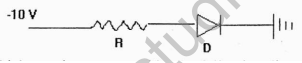9.which gate is represented by the following diagram?.10.The ratio of number of free electrons to holes ne/nh for two different materials A and B are 1 and <1 respectively. Name the type of semi conductor to which A and B belongs.
11.In half wave rectification , what is the output frequency if the input frequency is 50 hz. What is the output frequency of a full wave rectification for the same input frequency.
12. How can you relate drift velocity and mobility of an electron?
13. Show by the graph how does the current vary with the voltage change for a junction diode.
14. Why do semiconductors obey OHM’S law for only low fields?
15. Mention the factors upon which Tranconductance of a transistor depend.
16. For faster action which transistor is used and why?
17. What are input and output characteristics of a transistor? Draw the graphs.
Ans:
18. A germanium diode is preferred to a silicon one for rectifying small voltages. Explain why?
Ans: Because the energy gap for Ge ( Eg = 0.7 ev) is smaller than the energy gap for Si (Eg = 1.1ev ) . Moreover, the germanium diode is much more open to the danger of high temperature affect than silicon at high voltage.
19.Express by a truth table the output Y for all possible inputs A and B in the circuit shown below.20. Write the Boolean equation and truth table for the circuit shown below.What is the output when all the inputs are high?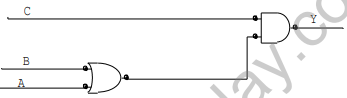21) Construct AND gate using NAND GATE and give its truth table.

23. For a common emitter amplifier, current gain = 50. If the emitter current is 6.6mA, calculate collector and base current. Also calculate current gain, when emitter is working as common base amplifier.

24.The base current is 100μA and collector current is 3mA.
a) Calculate the values of b, Ie, and a
b) A change of 20μA in the base current produces a change of 0.5mAin the collector current. Calculate ba.c.

25. In NPN transistor circuit, the collector current is 5mA. If 95% of the electrons emitted reach the collector region,what is the base current?
Ans: Here
Ic=95% of Ie = (95 / 100 ) Ie
Ie = (100 / 95) × 5 mA = 5.26mA
Ie= Ic+ Ib
Ib = 0.25 mA

26. In a transistor circuit shown the figure, the emitter current is 5mA and collector current 4.75 mA. Calculate the base current and the value of Rb.
27.A circuit symbol of a logic gate and two input wave forms A and B are shown.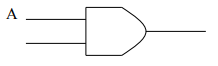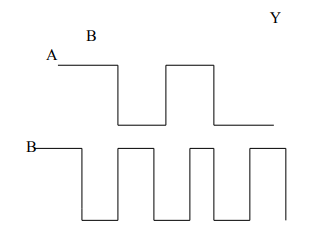a) Name the logic gate
b) Give the output wave form

28. The diode shown in the figure has a constant voltage drop of 0.5V at all currents and a maximum power rating of 100mW. What should be the value of resistance R connected in series,
for maximum current.?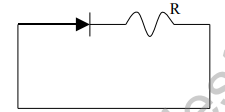29.For a transistor working as a common base amplifier, current gain is 0.96. If the emitter current is 7.2mA, then calculate the base current.

30. For a common emitter amplifier, the current gain is 70. If the emitter current is 8.8mA, calculate the collector and base current.

31.The base current of a transistor is 105 μA and collector current is 2.05 mA.
a) Deterine the value of b , Ie , and a
b) A change of 27 μA in the base current produces a change of 0.65 mA in the collector current . Find ba.c.

32. In a silicon transistor, a change of 7.89mA in the emitter current produces a change of 7.8 mA in the collector current. What change in the base current is necessary to produce an equivalent change in the collector current?

(1) Out of electrons and holes, which has higher mobility?

(2) Which special type of diode can act as a voltage regulator? Give the symbol of this diode and draw the general shape of its V-I characteristics.
(3) What do you mean by rectification?
(4) If a semiconductor has an intrinsic carrier concentration 1.04x1014/m3 of when doped with 1023/m3phosphorous atoms, calculate the concentration of hole/mat room temperature .
(5) In a common emitter circuit, if VCE is changed by 0.5 V, collector current Changes by 0.002 mA. What is the output resistance?
(6) Name the electrical circuits used to get smooth D.C. output from a rectified circuit.
(7) How does the energy gap of an intrinsic semiconductor vary when doped with a trivalent impurity?
(8) Draw energy band diagram of n-type semiconductor.
(9) A semiconductor has equal electron and hole concentration 6×108 /m3 .On doping with a certain impurity, electron concentration increases to 8×1012 /m3 .Identify the type of
semiconductor after doping.
(10) How does the dc current gain of a transistor change, if the width of the base region is increased?
(11) Why are photodiodes used preferably in reverse bias condition?

2 marks questions
(1) In the working of a transistor, emitter base junction is forward biased, while the collector base junction is reverse biased, why: To make transistor to act as an amplifier.
(2) In a transistor base is lightly doped and is a thin layer, why? To reduce the neutralization in the base emitter junction.
(3)What is the condition for the state of saturation of a transistor?
(4)Write the truth table for the following logic circuit shown in the figure below.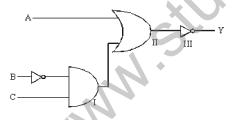3 marks questions
(1) Discuss how the OR gate is realized from the NOR gate.
(2) Why is the base region of a transistor usually made thin?In a common emitter mode of transisitor, d.c. current gain is 20, the emitter current is 7 mA. Calculate (i) base current,
and (ii) collector current.
(3) The input resistance of a silicon transistor is 665 Ω. Its base current is changed by 15 μA, which results in change of collector current by 2mA. This transistor is used as a common emitter amplifier with a load resistance of 5 k .
Calculate (i) current gain ,
(ii) transconductance gm, and (iii) voltage gain Av of the amplifier.
(4) Draw the energy band diagram of a p-type semiconductor. Distinguish between p-type and n-type semiconductor.
(5)Explain briefly with the help of a circuit, how V-I characteristics of a p-n junction diode are obtained in (a) Forward bias (b) Reverse bias. Draw the shape of curves obtained.
(6)In a common emitter transistor amplifier, the input resistance of a transistor is 100 ohm. On changing its base current by 10μA, the collector current increases by 2mA.If a load
resistance of 5kilo ohm is used in the circuit, calculate (a) current gain (b) voltage gain of the amplifier
(7) Two signals A, B as given below, are applied as input to
(i) AND (ii) NOR and
(iii) NANDgates. Draw the output wave-form in each case.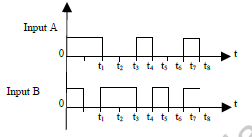5 marks questions
(1) Draw a circuit diagram to obtain the characteristics of a n-p-n transistor in common emitter configuration. Describe how you will obtain input and output characteristics.
Give shape of the curves.
(2) Explain the function of base region of a transistor. Draw a circuit diagram to study the input and output characteristics of NPN transistor in a common emitter (CE) configuration. Show these characteristics graphically. Explain how the current amplification factor is calculated from these characteristics.
(3) Explain the working of transistor as an oscillator with the help of a neat diagram.
(4) A student has to study the input and output characteristics of a n-p-n silicon transistor in the Common Emitter configuration. What kind of a circuit arrangement should she use for this purpose? Draw the typical shape of input characteristics likely to be obtained by her. What do we understand by the cut off, active and saturation states of the transistor? In which of these states does the transistor not remain when being used as a switch?
(5) Input signals A and B are applied to the input terminals of the ‘dotted box’ set-up shown here. Let Y be the final output signal from the box. Draw the wave forms of the signals labeled as C1 and C2 within the box, giving (in brief) the reasons for getting these wave forms. Hence draw the wave form of the final output signal Y. Give reasons for your choice. What can we state (in words) as the relation between the final output signal Y and the input signals A and B?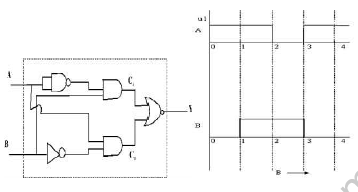CBSE Class 12 Physics HOTs Atoms And Nuclei CBSE Class 12 Physics HOTs Communication Systems Set C CBSE Class 12 Physics HOTs Optics Set A CBSE Class 12 Physics HOTs Optics Set B CBSE Class 12 Physics HOTs Optics Set C
 CBSE Class 12 Physics HOTs Electrostatic Potential and Capacitance
 CBSE Class 12 Physics HOTs Current Electricity
 CBSE Class 12 Physics HOTs Electromagnetic Induction and Alternating Current
 CBSE Class 12 Physics HOTs Electromagnetic Waves
 CBSE Class 12 Physics HOTs Wave Optics
 CBSE Class 12 Physics HOTs Dual Nature Of Matter And Radiations
 CBSE Class 12 Physics HOTs Conductor CBSE Class 12 Physics HOTs Electronic Devices Set A CBSE Class 12 Physics HOTs Electronic Devices Set B CBSE Class 12 Physics HOTs Semiconductor Electronics Materials and Devices and Simple Circuits
 CBSE Class 12 Physics HOTs Communication Systems

## More Study Material

### CBSE Class 12 Physics Chapter 14 Semiconductor Electronics Materials Devices and Simple Circuits HOTS

We hope students liked the above HOTS for Chapter 14 Semiconductor Electronics Materials Devices and Simple Circuits designed as per the latest syllabus for Class 12 Physics released by CBSE. Students of Class 12 should download the High Order Thinking Skills Questions and Answers in Pdf format and practice the questions and solutions given in above Class 12 Physics  HOTS Questions on daily basis. All latest HOTS with answers have been developed for Physics by referring to the most important and regularly asked topics that the students should learn and practice to get better score in school tests and examinations. Studiestoday is the best portal for Class 12 students to get all latest study material free of cost.

### HOTS for Physics CBSE Class 12 Chapter 14 Semiconductor Electronics Materials Devices and Simple Circuits

Expert teachers of studiestoday have referred to NCERT book for Class 12 Physics to develop the Physics Class 12 HOTS. If you download HOTS with answers for the above chapter daily, you will get higher and better marks in Class 12 test and exams in the current year as you will be able to have stronger understanding of all concepts. Daily High Order Thinking Skills questions practice of Physics and its study material will help students to have stronger understanding of all concepts and also make them expert on all critical topics. You can easily download and save all HOTS for Class 12 Physics also from www.studiestoday.com without paying anything in Pdf format. After solving the questions given in the HOTS which have been developed as per latest course books also refer to the NCERT solutions for Class 12 Physics designed by our teachers

#### Chapter 14 Semiconductor Electronics Materials Devices and Simple Circuits HOTS Physics CBSE Class 12

All HOTS given above for Class 12 Physics have been made as per the latest syllabus and books issued for the current academic year. The students of Class 12 can refer to the answers which have been also provided by our teachers for all HOTS of Physics so that you are able to solve the questions and then compare your answers with the solutions provided by us. We have also provided lot of MCQ questions for Class 12 Physics in the HOTS so that you can solve questions relating to all topics given in each chapter. All study material for Class 12 Physics students have been given on studiestoday.

#### Chapter 14 Semiconductor Electronics Materials Devices and Simple Circuits CBSE Class 12 HOTS Physics

Regular HOTS practice helps to gain more practice in solving questions to obtain a more comprehensive understanding of Chapter 14 Semiconductor Electronics Materials Devices and Simple Circuits concepts. HOTS play an important role in developing an understanding of Chapter 14 Semiconductor Electronics Materials Devices and Simple Circuits in CBSE Class 12. Students can download and save or print all the HOTS, printable assignments, and practice sheets of the above chapter in Class 12 Physics in Pdf format from studiestoday. You can print or read them online on your computer or mobile or any other device. After solving these you should also refer to Class 12 Physics MCQ Test for the same chapter

#### CBSE HOTS Physics Class 12 Chapter 14 Semiconductor Electronics Materials Devices and Simple Circuits

CBSE Class 12 Physics best textbooks have been used for writing the problems given in the above HOTS. If you have tests coming up then you should revise all concepts relating to Chapter 14 Semiconductor Electronics Materials Devices and Simple Circuits and then take out print of the above HOTS and attempt all problems. We have also provided a lot of other HOTS for Class 12 Physics which you can use to further make yourself better in Physics.

Where can I download latest CBSE HOTS for Class 12 Physics Chapter 14 Semiconductor Electronics Materials Devices and Simple Circuits

You can download the CBSE HOTS for Class 12 Physics Chapter 14 Semiconductor Electronics Materials Devices and Simple Circuits for latest session from StudiesToday.com

Can I download the HOTS of Chapter 14 Semiconductor Electronics Materials Devices and Simple Circuits Class 12 Physics in Pdf

Yes, you can click on the link above and download topic wise HOTS Questions Pdfs for Chapter 14 Semiconductor Electronics Materials Devices and Simple Circuits Class 12 for Physics

Are the Class 12 Physics Chapter 14 Semiconductor Electronics Materials Devices and Simple Circuits HOTS available for the latest session

Yes, the HOTS issued by CBSE for Class 12 Physics Chapter 14 Semiconductor Electronics Materials Devices and Simple Circuits have been made available here for latest academic session

How can I download the Class 12 Physics Chapter 14 Semiconductor Electronics Materials Devices and Simple Circuits HOTS

You can easily access the link above and download the Class 12 HOTS Physics Chapter 14 Semiconductor Electronics Materials Devices and Simple Circuits for each topic

Is there any charge for the HOTS with solutions for Chapter 14 Semiconductor Electronics Materials Devices and Simple Circuits Class 12 Physics

There is no charge for the HOTS and their answers for Chapter 14 Semiconductor Electronics Materials Devices and Simple Circuits Class 12 CBSE Physics you can download everything free

What does HOTS stand for in Class 12 Physics Chapter 14 Semiconductor Electronics Materials Devices and Simple Circuits

HOTS stands for "Higher Order Thinking Skills" in Chapter 14 Semiconductor Electronics Materials Devices and Simple Circuits Class 12 Physics. It refers to questions that require critical thinking, analysis, and application of knowledge

How can I improve my HOTS in Class 12 Physics Chapter 14 Semiconductor Electronics Materials Devices and Simple Circuits

Regular revision of HOTS given on studiestoday for Class 12 subject Physics Chapter 14 Semiconductor Electronics Materials Devices and Simple Circuits can help you to score better marks in exams

Are HOTS questions important for Chapter 14 Semiconductor Electronics Materials Devices and Simple Circuits Class 12 Physics exams

Yes, HOTS questions are important for Chapter 14 Semiconductor Electronics Materials Devices and Simple Circuits Class 12 Physics exams as it helps to assess your ability to think critically, apply concepts, and display understanding of the subject.